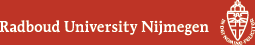## Geometry Seminar - Abstracts

### Talk

4 February 2016, 16:00 - 17:00, in HG00.310
Erdal Emsiz (Pontificia Universidad Catolica de Chile)
Difference equation for the Heckman-Opdam hypergeometric function and its confluent Whittaker limit

### Abstract

We will discuss explicit difference equations for the Heckman-Opdam hypergeometric function associated with root systems (a generalization of the Gauss hypergeometric function to various variables). Our method exploits the fact that for discrete spectral values on a (translated) cone of dominant weights the Heckman-Opdam hypergeometric function truncates in terms of Heckman-Opdam Jacobi polynomials. This permits us to derive/prove the desired difference equations in two steps: first for the discrete spectral values by performing a $q\to 1$ degeneration of a recently found Pieri formula for the celebrated Macdonald polynomials, and then for arbitrary spectral values upon invoking an analytic continuation argument borrowed from Rösler (based on known growth estimates for the Heckman-Opdam hypergeometric function that enable one to apply Carlson's theorem).

If time permits we will also mention analogous difference equation for the class-one Whittaker function diagonalizing the open quantum Toda chain associated with reduced root systems.

Based on joint work with Jan Felipe van Diejen (Universidad de Talca).